# 1- Detection Algorithm

• Image Classification：判断图片中是否包含某物。
• Classification with localization：判断图片是否包含某物，以及某物的边框。
• Detection：图片中包含多个属于不同类别的对象，识别出包含的所有对象，以及其边框。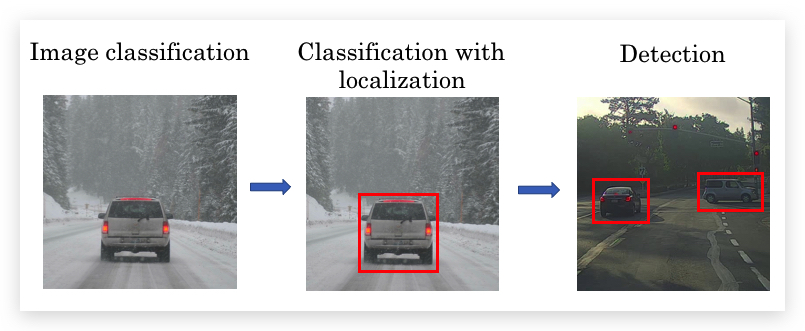## 1.1- Object Localization

1. bounding box• $$b_x$$: 物体中心点的x坐标
• $$b_y$$: 物体中心点的y坐标
• $$b_h$$: 矩形框的高度
• $$b_w$$: 矩形框的宽度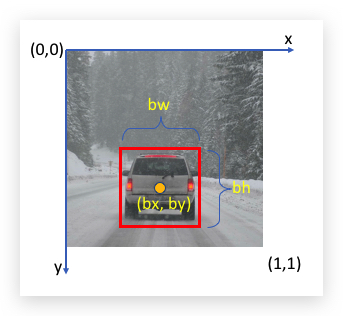1. Classification with localization

Classification问题，最终通过softmax输出one-hot向量，表示属于哪个类型的对象，比如一个路面识别四种类型（1-行人 2-车辆 3-摩托车 4-背景）的Classification的卷积网络如下：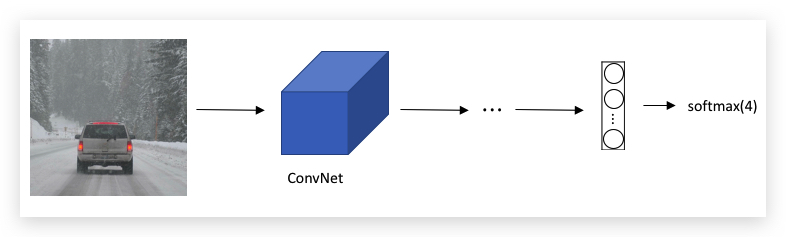Classification with localization则在上述基础上，增加bounding box坐标的输出：1. Defining the target label y

• $$p_c$$： 表示物体是否存在
• bounding box的四个参数：$$b_x, b_y, b_h, b_w$$
• 物体分类的one-hot向量

$\\left[ \\begin{matrix} 1 \\\\ 0.5 \\\\ 0.7 \\\\ 0.3 \\\\ 0.4 \\\\ 0 \\\\ 1 \\\\ 0 \\\\ 0 \\\\ \end{matrix} \\right]$

$\\left[ \\begin{matrix} 0 \\\\ ? \\\\ ? \\\\ ? \\\\ ? \\\\ ? \\\\ ? \\\\ ? \\\\ ? \\\\ \end{matrix} \\right]$

1. Loss function

• 如果$$\hat y$$里的$$p_c=1$$，则： $$L(\hat y,y)=(\hat y_1-y_1)^2+(\hat y_2-y_2)^2+\cdots+(\hat y_8-y_8)^2$$
• 如果$$\hat y$$里的$$p_c=0$$则仅需要计算$$p_c$$的偏差，其他分量不需要考虑： $$L(\hat y,y)=(\hat y_1-y_1)^2$$

## 1.2- Landmark Detection

Localization给出了bounding box的坐标，更一般的，可以让卷积网络学习识别关键点的坐标，这些关键点就是特征点（Landmark），这个过程即特征点检测（Landmark Detection）。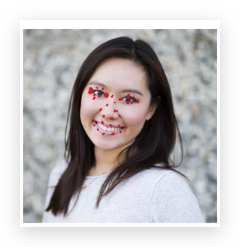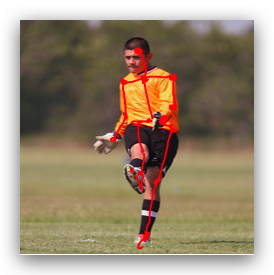Landmark Detection的应用：面部识别、动作捕捉、AR。

## 1.3- Object Detection

1. Closely cropped images 假如要做的Car Detection，首先用closely cropped images（即紧贴着对象裁剪的图片）训练一个卷积网络，可以识别closely cropped images是否是汽车图片。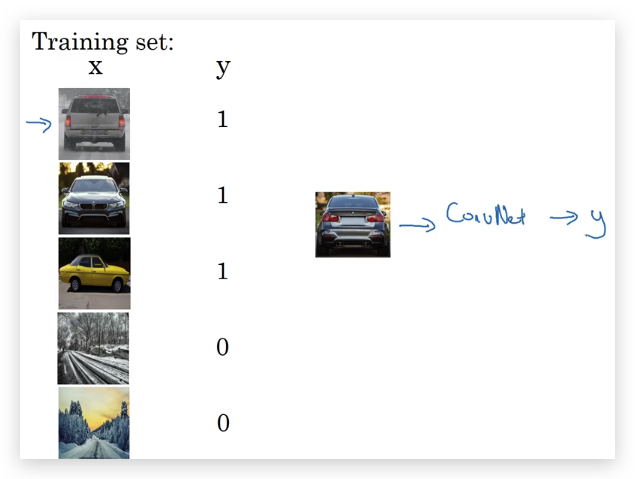1. Sliding windows detection

• 首先选取一个固定大小的矩形window，用这个window以一个步长在图片上从左到右，从上到下依次扫过。
• 每次window扫过的区域，使用之前根据closely cropped images训练好的卷积网络，识别区域内是否有目标对象。
• 不断增大window的大小，重复上面的操作。最后可能在某一个大小的window，在某个位置输出包含目标对象，这个位置的window就是目标对象的bounding box。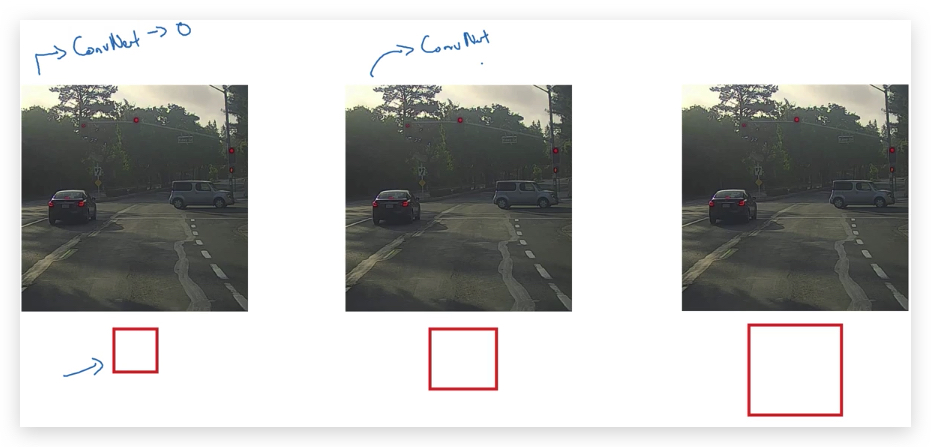Sliding windows的主要缺点：

• 计算量巨大，因为要用不同大小的window，对整个图片不同位置做检测。window的大小和步长的大小选择对计算量影响也很大。
• 如果window选择的太大、步长太大，定位的精度也很差。

## 1.4- Convolutional Implementation of Sliding Windows

1. Turning FC layer into Convolutional layer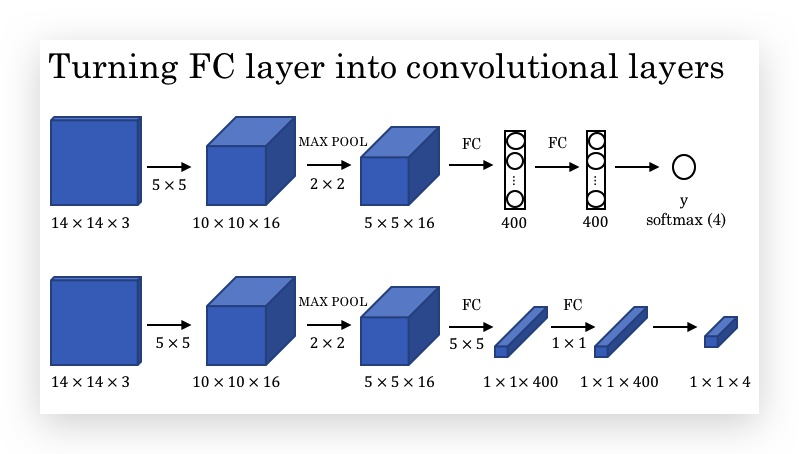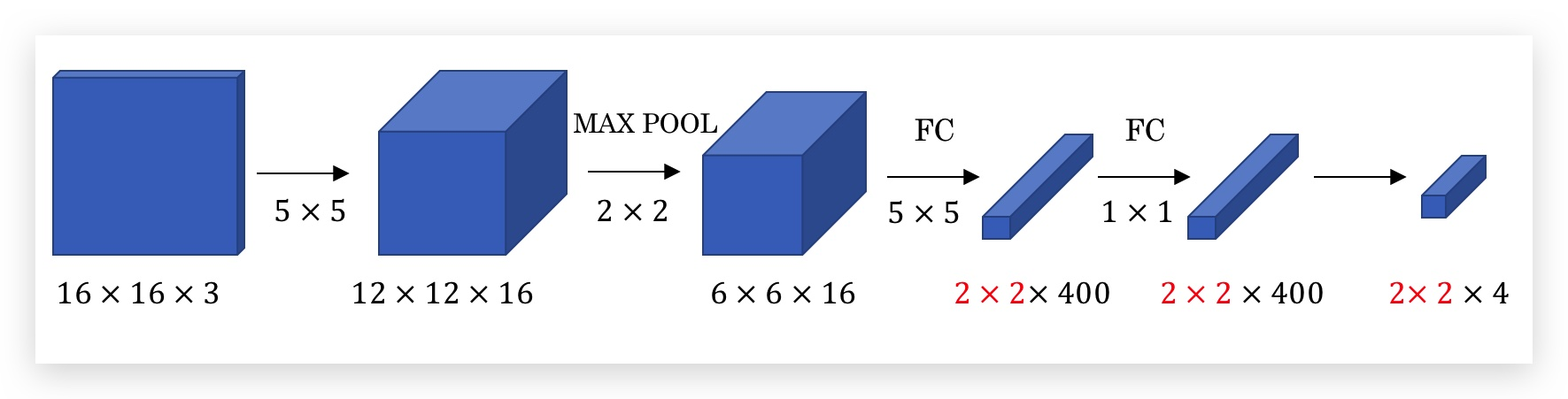1. Convolutional Implementation of Sliding Windows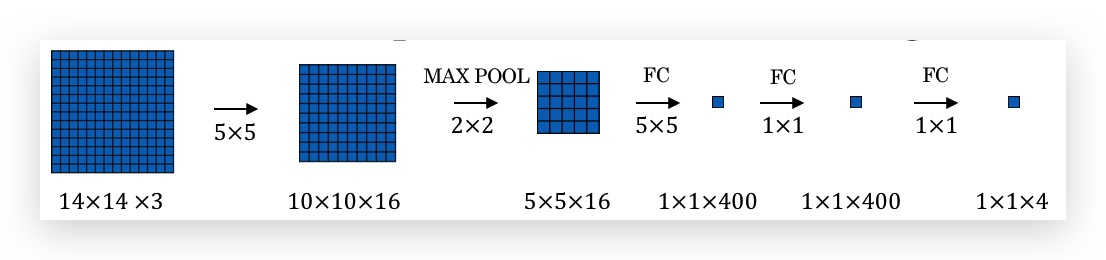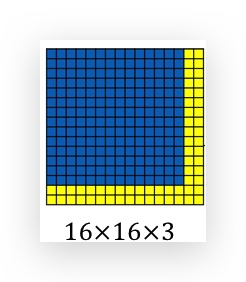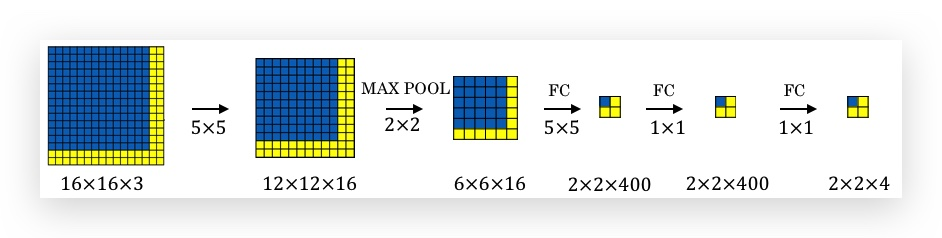• 卷积的计算，本身就是类似于slide window，不断的将filter以一个stride在原始图片上从左到右，从上到下滑动做卷积。而这个过程和单独slide好window交给Closely cropped images是一样的过程。
• ConvNet是稀疏连接的，比如2x2的输出结果，左上角的一个输出值仅受输入图片左上角14x14的区域影响
• 由于上面的原因，一方面ConvNet帮助共享了计算，并同时参与计算，而不是像sliding window那样串行的计算，另一方面又达到了和sliding window的效果一致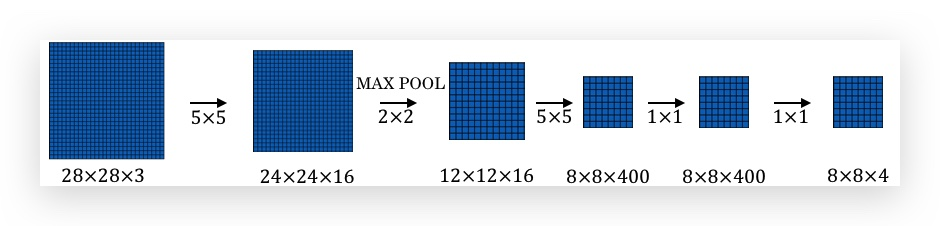## 1.5- Bounding Box Predictions

1. YOLO的基本过程：
• 没有sliding window，而是将图片拆分为若干个grid（比如示例中拆分为3x3，共9个grid；实际操作会拆分更多）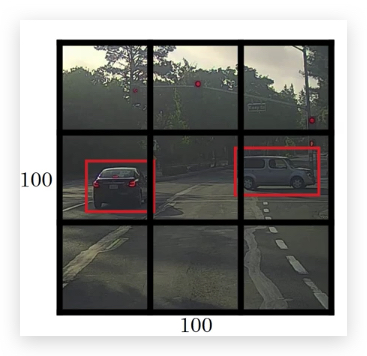• 对每个grid应用Classification with localization算法（前面章节提到的），训练数据和算法的输出包含了目标的bounding box坐标的向量，比如： $$\\left[ \\begin{matrix} p_c \\\\ b_x \\\\ b_y \\\\ b_h \\\\ b_w \\\\ c_1 \\\\ c_2 \\\\ c_3 \\\\ \end{matrix} \\right]$$
• 一共输出9个向量（和grid个数一样）
2. 关于bounding box的坐标：
• 目标是否存在于grid的标准是目标的中心点是否在grid内
• bounding box的坐标是以grid为参考的，而非整个图片。即将一个grid的左上角设置为(0,0)，右下角设置为(1,1)。
• bounding box的中心点坐标$$b_x$$和$$b_y$$的值必须是0到1之间，但高度$$b_h$$和宽$$b_w$$可以是比1大，说明超过了grid（事实上不超过1也可能是在grid之外），换句话说，高度和宽度是按照物体的实际高度和宽度来算的，不受grid的限制
• bounding box的大小不是固定的，而是直接由模型输出，模型可以以任何精度输出bounding box
3. YOLO算法是卷积实现

1. YOLO的优点：
• 和Classification with localization类似，直接输出bounding box，不受sliding window和步长的限制，更为精确。
• 卷积实现，仅进行一次CNN正向计算，计算量降低。

paper参考：You Only Look Once: Unified, Real-Time Object Detection Andrew Ng建议：YOLO的paper是最难的paper之一，阅读要有心理准备。

## 1.6- Intersection Over Union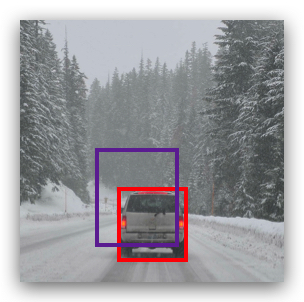$IoU = \frac{\text{Intersection}}{\text{Union}}$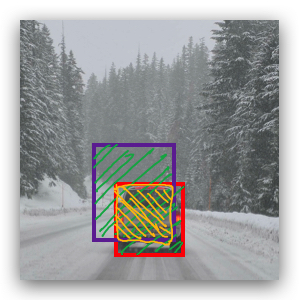IoU 的值介于0到1之间，越接近1表示目标的定位越准确，IoU惯例上用>=0.5表示“正确”定位的阈值，或根据情况设置这个阈值。

## 1.7- Non-max Suppression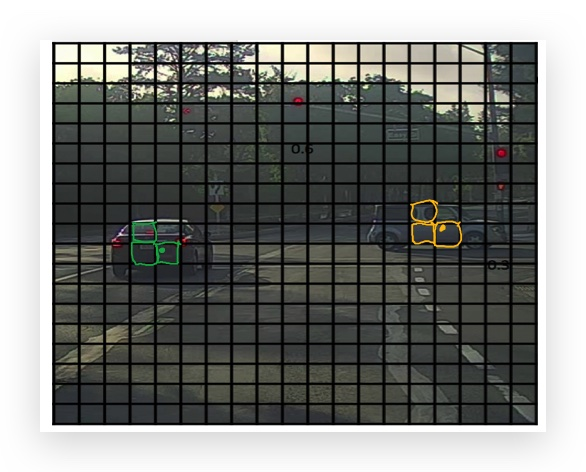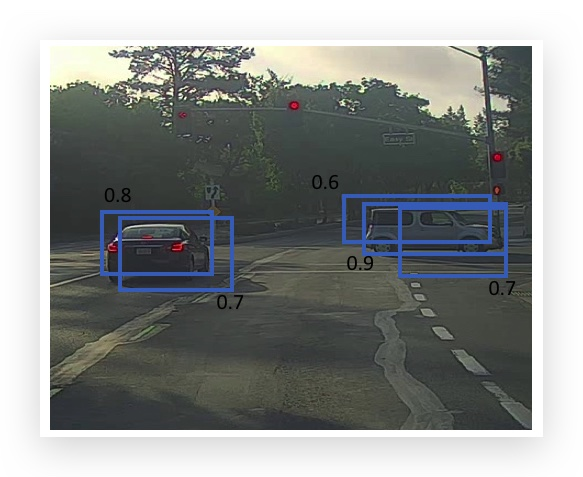1. 首先丢弃所有$$p_c$$值小于0.6（该阈值可调整）的grid。（$$p_c$$太小的，很可能是识别了一些边边角角，可以直接丢弃）
2. 对于所有剩余的grid
• 选取$$p_c$$值最大的grid A作为输出，即从剩余grid拿掉。
• 丢弃所有与A的IoU大于等于0.5（该阈值可调整）的grid。（这些很可能是与A相邻的，识别了同一个对象）
3. 重复第2步，直到没有剩余的grid为止。

1. 丢弃小于0.6的grid，这里没有要丢弃的
2. 选取最大的0.9的grid作为第一个目标的输出，并且将与其bounding box的IoU超过0.5的两个grid（即右边的两个0.6和0.7的）剔除。
3. 接下来再看剩余grid（只有左边0.7和0.8两个）里$$p_c$$值最大的grid，显然是0.8的grid，将其作为第二个目标的输出。0.7与其IoU超过0.5，被剔除。
4. 已无剩余的grid，并输出了两个目标的grid。

non-max的意思就是输出最大可能性的grid，并抑制与其有重叠的非最大值grid

## 1.8- Anchor Boxes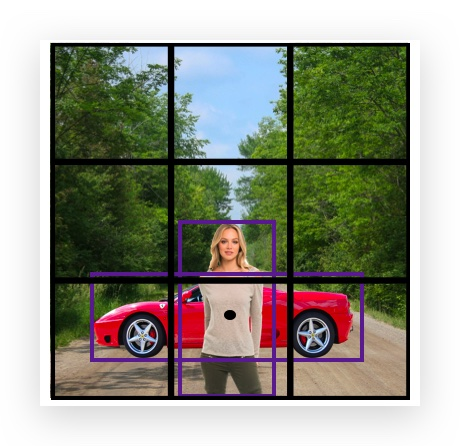$\\left[ \\begin{matrix} p_c \\\\ b_x \\\\ b_y \\\\ b_h \\\\ b_w \\\\ c_1 \\\\ c_2 \\\\ c_3 \\\\ \end{matrix} \\right]$

Anchor Boxes的思想是，预定义两个形状不同的称作Anchor Box的矩形。 将要预测的两个对象分别关联到两个Anchor Box上。（实践中，会定义多个Anchor Box，这里只用2个作说明 ）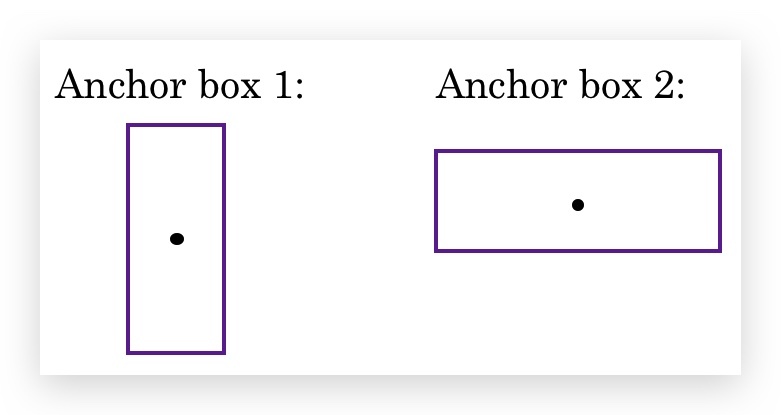• 原算法：训练图片中的每个目标，被分配到包含该物体中心点的grid里。
• Ancho Boxes：训练图片中的每个目标，被分配到包含该物体中心点的grid和与其bounding box有最大IoU的anchor box中。相当于增加了一个匹配维度，即（grid cell, anchor box）。
• 本例中，y的维度从3x3x8变成了3x3x2x8。

Anchor Box无法处理的情况：

• 如果设定了两个anchor box，但grid里面有3个物体，则也无法处理
• 如果是两个物体的anchor box一样，也无法处理。

## 1.9- YOLO Algorithm

$\\left[ \\begin{matrix} p_c \\\\ b_x \\\\ b_y \\\\ b_h \\\\ b_w \\\\ c_1 \\\\ c_2 \\\\ c_3 \\\\ p_c \\\\ b_x \\\\ b_y \\\\ b_h \\\\ b_w \\\\ c_1 \\\\ c_2 \\\\ c_3 \\\\ \end{matrix} \\right]$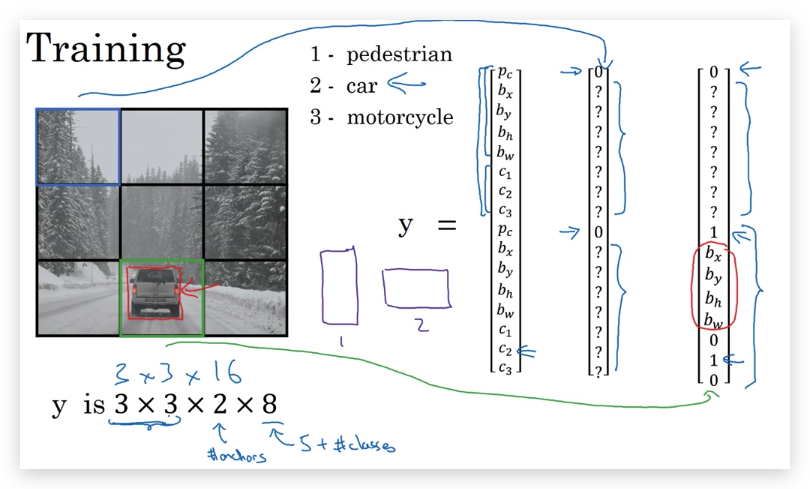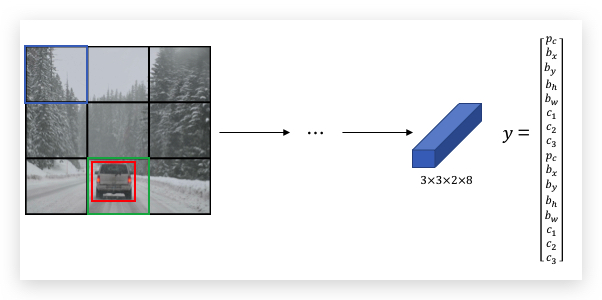## 1.10- Region Proposals

R-CNN（Regions with CNNs）的思想是，通过Segmentation algorithm算法（即产生最右面的色块图）找出候选区域，只对有可能出现目标的色块进行检测，输出label和bounding box。相比sliding window的逐行逐列，减少了检测量。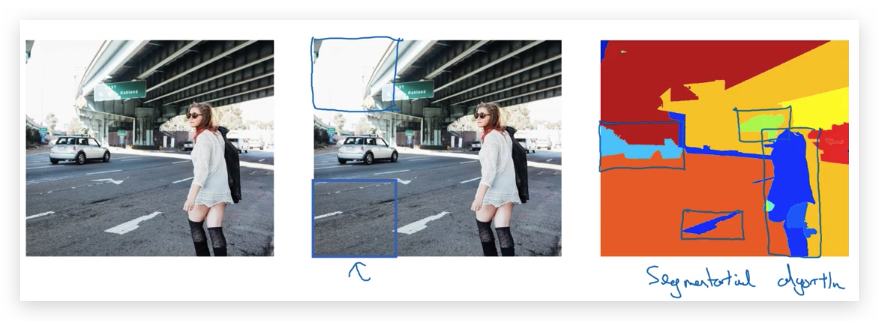R-CNN运算很慢，衍生出了改良算法：

• Fast R-CNN：使用Convolutional Implementation of Sliding Windows去识别候选区域。
• Faster R-CNN：使用CNN做候选区域的识别。

paper参考：

-------------------------